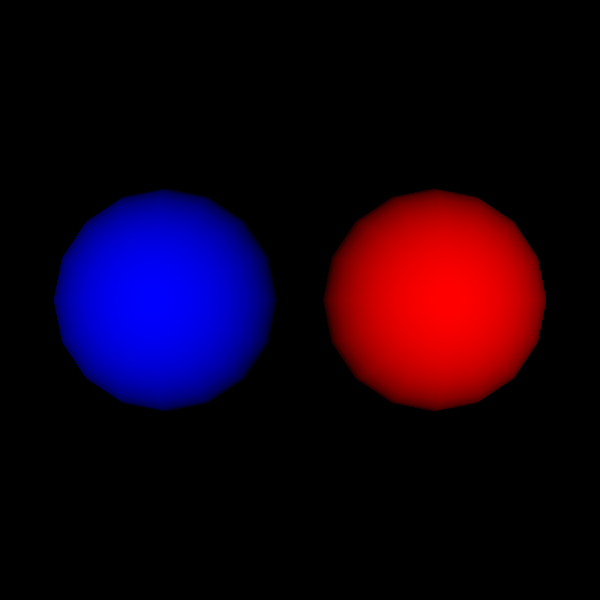# FURY sphere Actor#

This example shows how to use both primitive and vtkSource sphere actor.

```import numpy as np
from fury import window, actor
```

First thing, you have to specify centers and colors of the sphere

```centers = np.zeros([1, 3])
colors = np.array([0, 0, 1])
```

The below sphere actor is generated by repeating the sphere primitive.

```prim_sphere_actor = actor.sphere(centers, colors=colors, radii=5)
```

This time, we’re using vtkSphereSource to generate the sphere actor

```cen2 = np.add(centers, np.array([12, 0, 0]))
cols2 = np.array([1, 0, 0])

vtk_sphere_actor = actor.sphere(cen2, colors=cols2,

scene = window.Scene()
```

Adding our sphere actors to scene.

```scene.add(prim_sphere_actor)